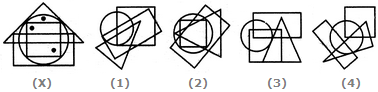# Non Verbal Reasoning - Dot Situation - Discussion

### Discussion :: Dot Situation - Section 1 (Q.No.5)

From amongst the figures marked (1), (2), (3) and (4), select the figure which satisfies the same conditions of placement of the dots as in figure (X).

5.

Select the figure which satisfies the same conditions of placement of the dots as in Figure-X.[A]. 1 [B]. 2 [C]. 3 [D]. 4

Explanation:

In fig. (X), one of the dots lies in the region common to the circle and the triangle only, another dot lies in the region common to the circle, the square and the triangle only and the third dot lies in the region common to the circle, the square and the rectangle only. In each of the figures (1) and (3) there is no region common to the circle and the triangle only. In fig. (4) there is no region common to the circle, the square and the rectangle only. Only fig. (2) consists of all the three types of regions.

 Sanjay said: (Mar 9, 2019) No, it is wrong because there is not having a common intersection of circle and triangle.

 Akash said: (Jul 18, 2019) Correct @Sanjay. The answer is 4.

 Chithu said: (Aug 29, 2019) How? The dot is present in all the shapes, Therefore 1 and 2 both are correct.

 Nischal said: (Jul 4, 2020) 1 is the correct answer.

 Rohan said: (Oct 31, 2020) The correct answer is 4.